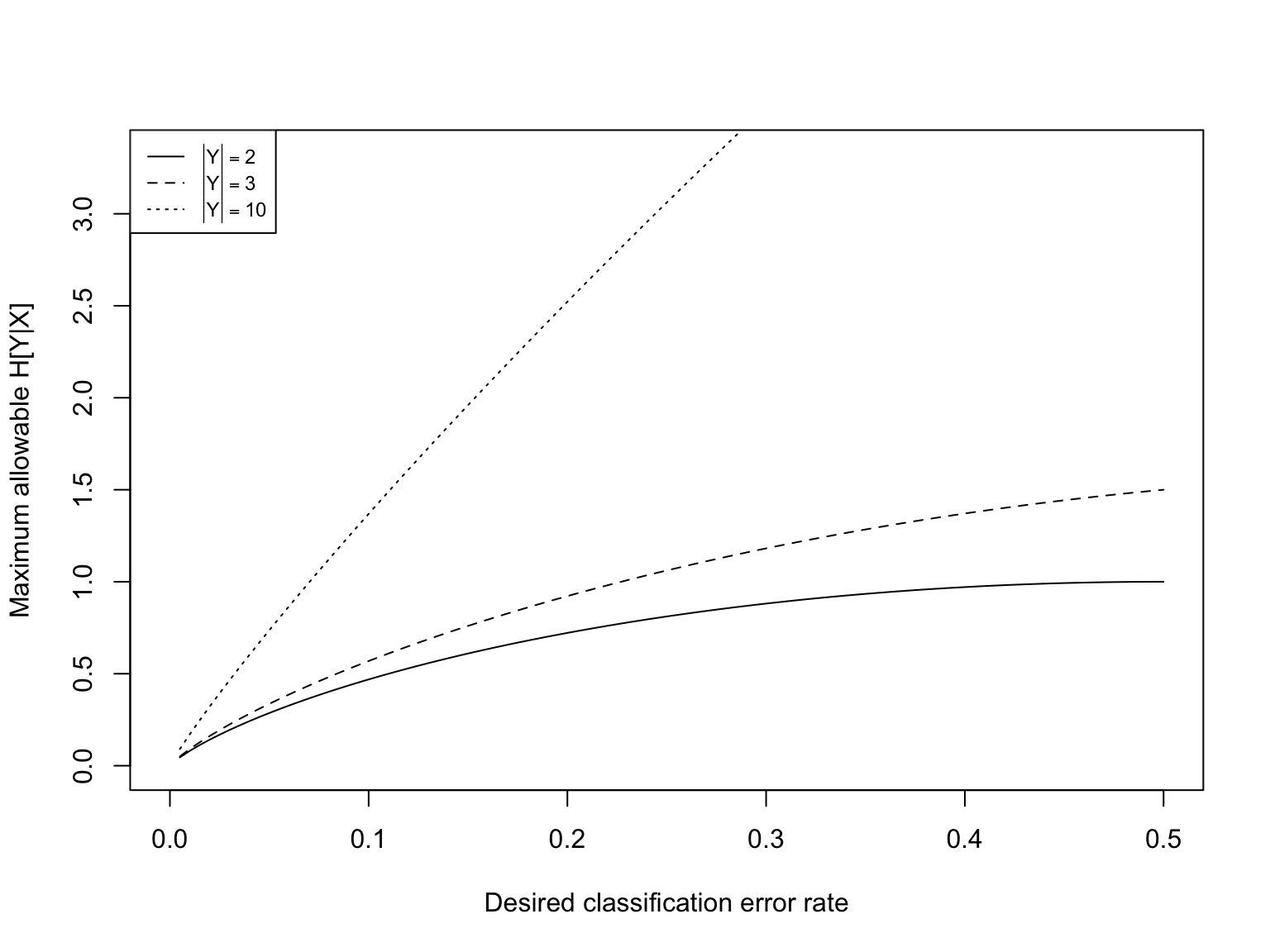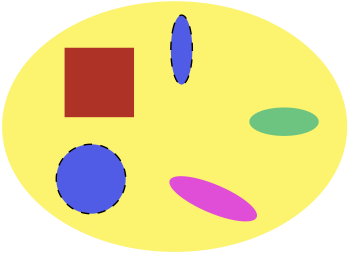# Information Theory III — Information for Prediction

Lecture 17 (23 October 2019)

$\newcommand{\Expect}{\mathbb{E}\left[ #1 \right]}$

# Information for Prediction

• We’ve seen the basic ideas of information theory:
• How much information/uncertainty is in one random variable ($$H[X]$$)
• How much information/uncertainty is in two RVs ($$H[X,Y]$$)
• How much information/uncertainty is left after conditioning ($$H[Y|X]$$)
• How much is uncertainty reduced by conditioning ($$H[Y] - H[X|Y]$$)
• How much information does one variable give about another ($$I[X;Y]=H[Y]-H[X|Y]$$)
• And conditional versions of all these
• We’ve seen how to use this for feature selection
• Rank features $$X_1, \ldots X_p$$ by $$H[Y|X_i]$$ or $$I[X_i;Y]$$
• Picking collects of features by evaluating $$I[Y; X_1, \ldots X_q]$$
• Now: What would success look like?
• Good features
• Good synthetic features

# Predictive Information

• We observe $$X$$ (possibly multivariate) and want to predict $$Y$$
• Conditioning on $$X$$ gives us some amount of information about $$Y$$ $I[X;Y] = H[Y] - H[Y|X]$
• Our prediction is a function of $$X$$, say $$\hat{Y}(X)$$
• Conditioning on $$\hat{Y}$$ never gives us more information: $I[\hat{Y};Y] = H[Y] - H[Y|\hat{Y}] \leq I[X;Y]$
• Because: (i) Conditioning never increases entropy, $$H[Y|X, f(X)] \leq H[Y|f(X)]$$
• But (ii) Conditioning on $$X$$ and $$f(X)$$ is the same as conditioning on $$X$$, so $$H[Y|X, f(X)] = H[Y|X]$$
• So $$H[Y|f(X)] \geq H[Y|f(X), X] = H[Y|X]$$
• This is (one form of) the data-processing inequality
• $$I[X;Y]$$ is the predictive information $$X$$ has about $$Y$$ and limits how good any prediction using $$X$$ can be

# Predictive Information vs. Accuracy

• For discrete $$Y$$, say $$M$$ (for “mistake”) $$=1$$ if $$Y\neq \hat{Y}$$ and $$=0$$ if $$Y=\hat{Y}$$
• Then one can show (see backup) that $H[Y|X] \leq H[M] + \Pr(M=1)\log{(|\mathcal{Y}|-1)}$
• Perfect classification ($$\Pr(M=1) = 0$$) implies $$H[Y|X] = 0$$
• RHS is an increasing function of $$\Pr(M=1)$$ so higher $$H[Y|X] \Rightarrow$$ higher inaccuracy, no matter what method we use# Predictive Information vs. Accuracy

• Upshot: $$H[Y|X]$$ lower bounds classification accuracy
• Even if we just care about accuracy, we should want to maximize information!

# Reducing the Features

• Suppose we look not at $$X$$ but $$T=\tau(X)$$
• Could be just picking out some dimensions of a multivariate $$X$$
• Could be applying transformations
• Could be creating new features out of old ones (as in PCA)
• Data-processing inequality says: $I[T;Y] \leq I[X;Y]$
• When will $$I[T;Y] = I[X;Y]$$?

# Sufficiency

• Re-write mutual informations: $I[X;Y] = \sum_{x}{p(x) \sum_{y}{p(y|x) \log{\frac{p(y|x)}{p(y)}}}}$ and $I[T;Y] = \sum_{t}{p(t) \sum_{y}{p(y|t)\log{\frac{p(y|t)}{p(y)}}}}$
• Suppose that $$\tau(x) = \tau(x^{\prime}) \Rightarrow p(y|x) = p(y|x^{\prime})$$
• Then for any $$x$$ with $$\tau(x) = t$$, $p(y|t) = p(y|x)$
• In more symbols, if $$x\in\tau^{-1}(t)$$ then $$p(y|t) = p(y|x)$$
• Why is this true?
• Use this to group terms in the $$x$$ sum for $$I[X;Y]$$: $\begin{eqnarray} I[X;Y] & = & \sum_{x}{p(x) \sum_{y}{p(y|x) \log{\frac{p(y|x)}{p(y)}}}}\\ & = & \sum_{t}{\sum_{x \in \tau^{-1}(t)}{p(x)\sum_{y}{p(y|x)\log{\frac{p(y|x)}{p(y)}}}}}\\ & = & \sum_{t}{\sum_{x \in \tau^{-1}(t)}{p(x) \sum_{y}{p(y|t)\log{\frac{p(y|t)}{p(y)}}}}}\\ & = & \sum_{t}{p(t) \sum_{y}{p(y|t)\log{\frac{p(y|t)}{p(y)}}}}\\ & = & I[T;Y] \end{eqnarray}$
• We say that $$T=\tau(X)$$ is sufficient for predicting $$Y$$ (from $$X$$)

# Sufficiency cont’d.

• Suppose that $\rho(x) = \rho(x^{\prime}) ~ \Leftrightarrow ~ p(y|x) = p(y|x^{\prime})$
• Then $$R=\rho(X)$$ will be sufficient
• And for any other sufficient $$T$$, $$R=g(T)$$ for some function $$g$$
• $$H[R] \leq H[T]$$ for any other sufficient $$T$$
• $$R$$ is minimal sufficient
• The way $$\rho$$ divides up $$X$$ is a statistical relevance basis (for predicting $$Y$$) (Salmon 1971, 1984; Shalizi and Crutchfield 2002)
• “Distinctions that make a difference”# From Sufficiency to the Information Bottleneck

• We can’t compress below $$R$$ without losing some predictive information
• What if we’re willing to give up some predictive information?
• Pick $$\beta > 0$$ and do $\max_{\tau}{I[Y;\tau(X)] - \beta H[\tau(X)]}$ or $\max_{\tau}{I[Y;\tau(X)] - \beta I[\tau(X);X]}$
• In words: Our benefit is the predictive information, our cost is the memory of the features
• $$\beta$$ is the price at which we trade predictive information against memory
• Lagrange multipliers: equivalent to maximizing $$I[Y;\tau(X)]$$ with a constraint on $$I[\tau(X);X]$$
• $$T=\tau(X)$$ is called the bottleneck variable
• Searching for this optimal $$\tau$$ (given $$\beta$$) is the information-bottleneck method (Tishby, Pereira, and Bialek 1999)

# Why This Matters

• Sometimes, we can explicitly work out the bottleneck or even the sufficient statistic
• Sometimes, it gives us a benchmark to evaluate against
• What’s $$I[Y;T]$$ for our favorite transformation of $$T$$ of the features?
• What’s $$H[T]$$ as compared to $$H[X]$$? How much information loss are we tolerating to get that compression?
• Sometimes it just inspires how we select features, or synthesize new features

# Dimension Reduction with a Target Variable

• Start with $$p$$-dimensional feature vector $$X$$
• Consider functions $$\tau$$ which map $$X$$ down to $$q$$-dimensional vectors $$T$$
• Maybe constrained, e.g., only functions linear in $$X$$
• Still want to maximize $$I[Y;T]$$, or maybe a bottlenecked version
• If calculating $$I[Y;T]$$ is too hard, or not quite right for the job, look at prediction error

# Dimension Reduction without a Target Variable

• Start with $$p$$-dimensional feature vector $$X$$
• Consider functions $$\tau$$ which map $$X$$ down to $$q$$-dimensional vectors $$T$$
• Think about how we’d reconstruct $$X$$ from $$T$$, $$\hat{X}(T)$$
• Maximize $$I[X;\hat{X}(T)]$$ with constraint on $$I[T;X]$$
• Again, can swap in minimizing prediction error if you want
• This is basically what we did in PCA!

# word2vec

• Start with words being binary features, $$p\approx$$ number of entries in the dictionary
• i.e., which word do we see at this position?
• Try to predict this word from neighboring words
• A huge problem!
• Map each word in to a vector of dimension $$q \ll p$$, maybe say $$q=700$$
• Adjust the mapping of words to vectors to maximize predictive information
• Experimentally, works better than PCA on the bag-of-word vectors
• But a lot more costly computationally (and in actual \$)

# Clustering

• Start with high-dimensional feature vectors $$X$$
• Now map them to a discrete set of categories, say $$k$$ of them
• $$I[\tau(X);X] \leq \log{k}$$ (why?)
• Try to maximize $$I[X;\hat{X}(T)]$$
• Or, if that’s too hard, some measure of the error of recovering $$X$$ from $$T$$
• This is clustering, and we’ll look at it for the next few lessons

# Summing up

• $$I[X;Y]$$ tells us about how well any method using $$X$$ can predict $$Y$$
• Sufficient statistics maximally compress $$X$$ without losing predictive information
• The information bottleneck method tells us about how to trade off compression against predictive information
• Dimension reduction $$\approx$$ looking for a continuous bottleneck
• Clustering $$\approx$$ looking for a discrete bottleneck

# Backup: Information for Continuous Variables

Replace sums with integrals as needed.

• $$X$$ and $$Y$$ both continuous: $\begin{eqnarray} I[X;Y] & = & \int{p(x,y) \left(\log{\left(\frac{p(x,y)}{p(x)p(y)}\right)}\right) dx dy}\\ & = & \int{p(x) \left(\int{p(y|x) \log{\left(\frac{p(y|x)}{p(y)}\right)} dy}\right) dx} \end{eqnarray}$ with $$p$$ being the pdf everywhere

• $$X$$ continuous, $$Y$$ discrete: $I[X;Y] = \int{p(x) \left(\sum_{y}{p(y|x) \log{\left(\frac{p(y|x)}{p(y)}\right)}}\right) dx}$ with $$p(x)$$ being the pdf but $$p(y|x)$$, $$p(y)$$ being the (conditional) pmf

• In any case:
• $$I[X;Y] = I[Y;X]$$
• $$I[X;Y] \geq 0$$
• $$I[X;Y] = 0$$ iff $$X$$ and $$Y$$ are independent
• $$I[f(X);Y] \leq I[X;Y]$$, with equality iff $$f(x) = f(x^{\prime}) \Rightarrow p(y|x) = p(y|x^{\prime})$$ (i.e., sufficiency)

# Backup: Conditional entropy and classification accuracy

$\begin{eqnarray} H[M|Y,\hat{Y}] & = & 0\\ H[Y|\hat{Y}] & = & H[Y|\hat{Y}] + H[M|Y,\hat{Y}]\\ & = & H[Y,M|\hat{Y}]\\ & = & H[M|\hat{Y}] + H[Y|M,\hat{Y}]\\ & = & H[M|\hat{Y}] + \Pr(M=0)H[Y|M=0,\hat{Y}] + \Pr(M=1)H[Y|M=1,\hat{Y}]\\ & \leq & H[M] + \Pr(M=0)H[Y|M=0,\hat{Y}] + \Pr(M=1)H[Y|M=1,\hat{Y}]\\ & = & H[M] + \Pr(M=0)\cdot 0 + \Pr(M=1) H[Y|M=1,\hat{Y}]\\ & = & H[M] + \Pr(M=1) H[Y|M=1,\hat{Y}]\\ & \leq & H[M] + \Pr(M=1)\log{(|\mathcal{Y}|-1)} \end{eqnarray}$

because if $$M=1$$, we know $$Y\neq \hat{Y}$$ and there are at most $$|\mathcal{Y}|-1$$ values $$Y$$ could have

Finally, remember that $$H[Y|\hat{Y}(X)] \geq H[Y|X]$$

This result is called Fano’s inequality

• Originally about recovering the true message ($$Y$$) from a noisy signal ($$X$$)
• For $$Y$$ uniformly distributed on $$\mathcal{Y}$$, Fano’s inequality implies $\Pr(M=1) \geq 1 - \frac{I[X;Y] + \log{2}}{\log{|\mathcal{Y}|}}$
• (Can you show this?)
• Fano’s inequality out to have many, many uses in prediction and estimation (Scarlett and Cevher, n.d.)
• Prediction: Obvious
• Estimation: Think of the parameter as the message ($$Y$$) we’re trying to recover from the noisy signal of the data set ($$X$$)

# Backup: Sufficiency and the Bottleneck, Take 2 (Shalizi and Crutchfield 2002)

• Initial bottleneck problem: $\max_{\tau}{I[Y;\tau(X)] - \beta H[\tau(X)]}$
• Willing to give up $$1$$ bit of predictive information if it saves at least $$\beta$$ bits of memory
• Lagrange multiplier form: $\max_{\tau}{I[Y;\tau(X)]} ~ \mathrm{subject\ to}~ H[\tau(X)] \leq c$
• Equivalently: compressing by 1 bit is only worthwhile if it reduces predictive information by $$\leq 1/\beta$$ bits $\min_{\tau}{H[\tau(X)] - (1/\beta) I[Y;\tau(X)]}$
• As $$\beta \rightarrow\infty$$, we become less and less willing to give up any predictive information
• Lagrange-multiplier form: $\min_{\tau}{H[\tau(X)]} ~ \mathrm{subject\ to} ~ I[Y;\tau(X)] \geq c^{\prime}$
• The limit $$\beta=\infty$$ is the minimal sufficient statistic / statistical relevance basis: $\min_{\tau}{H[\tau(X)]} ~\mathrm{subject\ to} ~ I[\tau(X);Y]=I[X;Y]$

# Backup: word2vec in a little more detail

• Each word $$w$$ corresponds to a vector $$v_w$$
• Each “context” = window of $$k$$ words around the focal word corresponds to a vector $$v_c$$
• Try to maximize $\max_{v_c, v_d}{\sum_{\mathrm{word}~ w ~\mathrm{appears\ in\ context} ~c}{\log{\frac{e^{v_c \cdot v_w}}{\sum_{c^{\prime}}{e^{v_{c^{\prime}} \cdot v_w}}}}}}$
• Words which appear in similar contexts should get similar vectors
• The actual maximization is too hard, so the word2vec software does something easier but related (Goldberg and Levy 2014)
• … which turns out to be (implicitly) factorizing a matrix of pointwise mutual informations between words and contexts (Levy and Goldberg 2014)